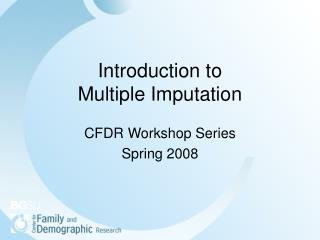DownloadDownload PresentationIntroduction to Multiple Imputation

# Introduction to Multiple Imputation

Télécharger la présentation## Introduction to Multiple Imputation

- - - - - - - - - - - - - - - - - - - - - - - - - - - E N D - - - - - - - - - - - - - - - - - - - - - - - - - - -
##### Presentation Transcript

1. Introduction to Multiple Imputation CFDR Workshop Series Spring 2008

2. Outline • Missing data mechanisms • What is Multiple Imputation? • SAS Proc MI, Proc MIANALYZE • Stata ICE, MICOMBINE • SAS IVEware • What’s the diff? • Problems with categorical imputation

3. Missing data mechanisms • Missing Completely At Random (MCAR) • The probability of missingness doesn't depend on anything. • Missing At Random (MAR) • The probability of missingness does not depend on the unobserved value of the missing variable, but it can depend on any of the other variables in your dataset • Not Missing at Random (NMAR) • The probability of missingness depends on the unobserved value of the missing variable itself

4. What is Multiple Imputation? • Imputation • Make M=3 to 10 copies of incomplete data set filling in with conditionally random values • Analyses • Of each data set separately • Pooling • Point estimates. Average across M analyses • Standard errors. Combine variances .

5. 1. Imputation: Multiple Copies of Dataset

6. Three steps • Imputation • Make M=2 to 10 copies of incomplete data set filling in with conditionally random values • Analyses • Of each data set separately • Pooling • Point estimates. Average across M analyses • Standard errors. Combine variances .

7. What is MI? • STATA • based on each conditional density • chained equations • SAS • joint distribution of all the variables • assumed multivariate normal distribution • SAS IVEware • same as Stata, more options.

8. Stata Example • ICE to impute • Regression commandsmay be logistic, mlogit, ologit, or regress. • MICOMBINE to analyze and combine the results. • Supported regression cmds are clogit, cnreg, glm, logistic, logit, mlogit, ologit, oprobit, poisson, probit, qreg, regress, rreg, stcox, streg, or xtgee. • Easy to use, nice documentation

9. SAS example

10. Step 1: Proc MI • Typical syntax: proc mi data=mi_example out=outmi seed=1234; var Oxygen RunTime RunPulse; run;

11. Step 2: Run Models procreg data=outmi outest=outreg covout noprint; model Oxygen = RunTime RUnPulse; by _Imputation_; run; Note that the regression output is stored as dataset “outreg” Proc’s= Reg, Logistic, Genmod, Mixed, GLM

12. Parameter Estimates & Covariance Matrices procprint data=outreg(obs=8); var _Imputation_ _Type_ _Name_ Intercept RunTime RunPulse; run;

13. Step 3. Proc Mianalyze procmianalyze data=outreg; modeleffects Intercept RunTime RunPulse; run;

14. Irritating Parameter Est. & Covariance Matrices • Syntax depends on what procedure you used in previous step: • proc mianalyze data=parmcov; (or) • proc mianalyze parms=parmsdat covb=covbdat; (or) • proc mianalyze parms=parmsdat xpxi=xpxidat; PROC’s: reg, genmod, logit, mixed, glm.

15. SAS IVEware: 4 Components 1. IMPUTE -- nice options. 2. DESCRIBE estimates the population means, proportions, subgroup differences, contrasts and linear combinations of means and proportions. A Taylor Series approach is used to obtain variance estimates appropriate for a user specified complex sample design. 3. REGRESS fits linear, logistic, polytomous, Poisson, Tobit and proportional hazard regression models for data resulting from a complex sample design. 4. SASMOD allows users to take into account complex sample design features when analyzing data with several SAS procedures. SAS PROCS can be called:CALIS, CATMOD, GENMOD, LIFEREG, MIXED, NLIN, PHREG, and PROBIT.

16. IVEware Impute IMPUTE assumes the variables in the data set are one of the following five types: (1) continuous (2) binary (3) categorical (polytomous with more than two categories) (4) counts (5) mixed The types of regression models used are linear, logistic, Poisson, generalized logit or mixed logistic/linear, depending on the type of variable being imputed.

17. SAS IVEware: 4 Components 1. IMPUTE -- nice options. 2. DESCRIBE estimates the population means, proportions, subgroup differences, contrasts and linear combinations of means and proportions. A Taylor Series approach is used to obtain variance estimates appropriate for a user specified complex sample design. 3. REGRESS fits linear, logistic, polytomous, Poisson, Tobit and proportional hazard regression models for data resulting from a complex sample design. 4. SASMOD allows users to take into account complex sample design features when analyzing data with several SAS procedures. SAS PROCS can be called:CALIS, CATMOD, GENMOD, LIFEREG, MIXED, NLIN, PHREG, and PROBIT.

18. A Few Issues • Do I impute the dependent variable? • Which model has more information? The imputation model or the analyst model? • How many imputations do I need to do? • Can I impute in one language and analyze in another? • How do I get summary statistics such as R squared? • Can I do this in SPSS? • Where do I go with questions?

19. Thanks Next up: “COLLATERAL CONSEQUENCES OF VIOLENCE IN DISADVANTAGED NEIGHBORHOODS” Dr. David HardingWednesday, February 13,Noon - 1:00 pm Accessing and Analyzing Add Health DataInstructor:  Dr. Meredith PorterMonday, February 25, 12:00-1:00 pm